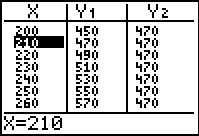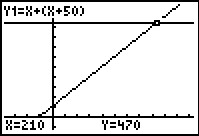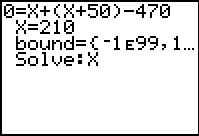# Activities

••• ##### Subject Area

• Math: Middle Grades Math: Problem Solving

• ##### Author6-8

45 Minutes

• ##### Device
• TI-73 Explorer™
• TI-Navigator™

## Four Ways to Get There#### Activity Overview

In this activity, students will use a variety of features of the TI-73 Explorer™ to represent problem situations. Students will look at problems algebraically, graphically, verbally, and numerically.

#### Key Steps

•Students will use a variety of features of the TI-73 to represent problem situations. Students will look at problems algebraically, graphically, verbally, and numerically.

First, students will enter the two sides of an equation into Y1 and Y2 and solve numerically using a table.

•Next, students will look at the same set of equations on a graph and find the intersection point using the Trace feature.

•Finally, students will use the Solver feature of the TI-73 to find a numerical solution exactly. Students will see that the same solution is obtained through the different methods and will begin to discuss some of the advantages and disadvantages to each method.